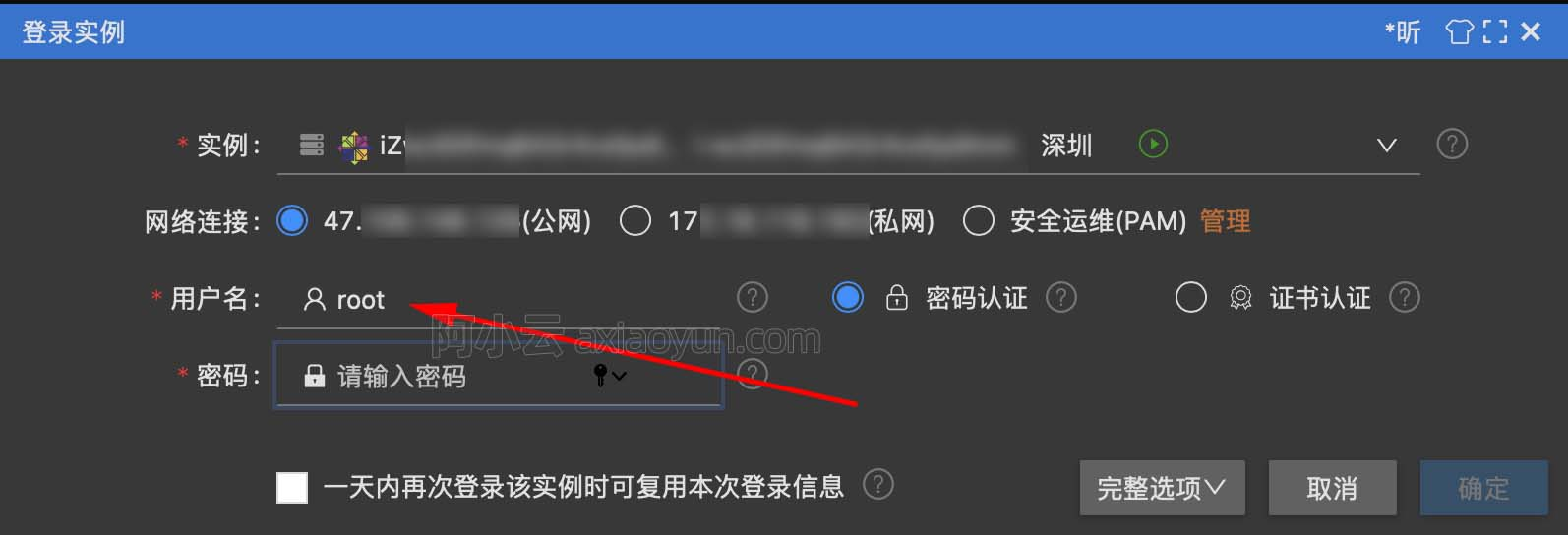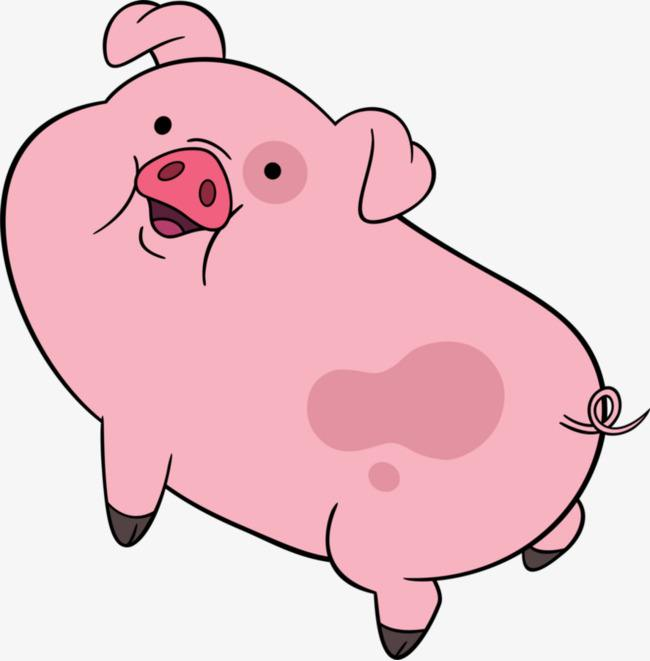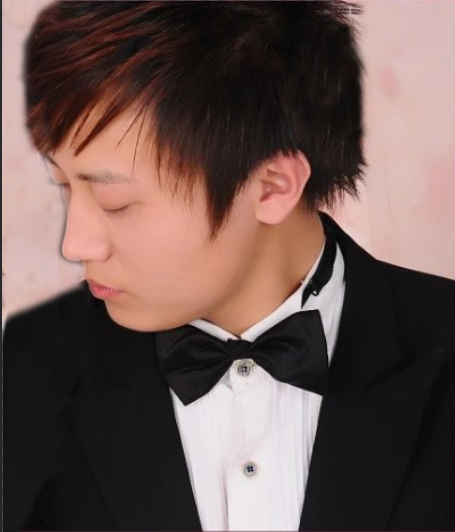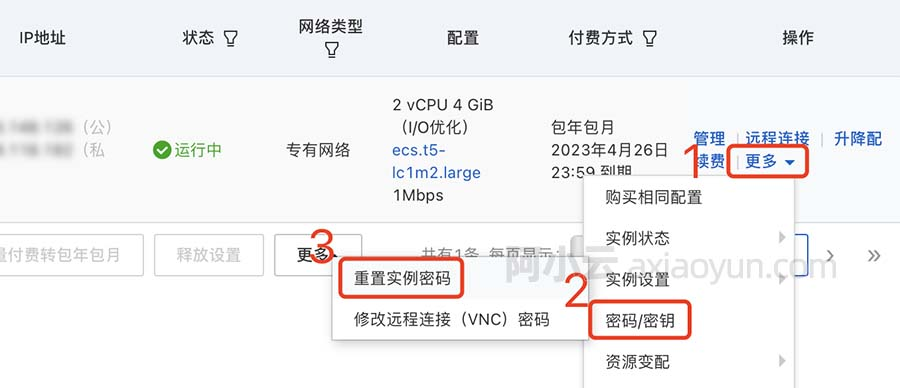# 使用图像文字识别技术获取失信黑名单

+关注继续查看test.png

In : import Image

In : import pytesseract

In : image = Image.open('/home/****/shixin/test.png')

In : image = image.convert('L')

In : text = ''.join(pytesseract.image_to_string(image,, config='-psm 6'))

In : print text

Zol l 〕西中执字第

1. 图像预处理

（1）灰度化

（2）二值化

def binarizing(image, threshold):    pixdata = image.load()    w, h = image.size    for y in range(h):        for x in range(w):            if pixdata[x, y] < threshold:                pixdata[x, y] = 0            else:                pixdata[x, y] = 255    return image2. 图像切割为单元格3. 单元格分类

4. 单元格分割为字符（1）判断是否是左右结构的汉字

（2）判断是否是左中右结构的汉字

（3）判断是否是左中中右结构的汉字

tmp_img_l = clear_image(image.crop((white_line[index - 1] + 1, 0, white_line[index], h))) tmp_img_r = clear_image(image.crop((white_line[index] + 1, 0, white_line[step_1], h))) tmp_w_l, tmp_h_l = tmp_img_l.size tmp_w_r, tmp_h_r = tmp_img_r.size word_image = clear_image(image.crop((white_line[index - 1] + 1, 0, white_line[step_1], h))) if ((tmp_h_r > 9 and tmp_h_l > 9) or (tmp_w_l < 4 or tmp_w_r < 4)) and parse_character(word_image, word_data):    for tmp_index in range(index, step_1):        tmp_white_line[tmp_index] = None else:    for tmp_index in range(step_1 + 1, len(white_line)):        if white_line[tmp_index] - white_line[tmp_index - 1] > 1:            step_2 = tmp_index            if None not in tmp_white_line[index - 1: step_2] and white_line[step_2] - white_line[index - 1] == 12:                tmp_img = clear_image(image.crop((white_line[index - 1] + 1, 0, white_line[step_2], h)))                tmp_w, tmp_h = tmp_img.size                if tmp_h > 10:                    for tmp_index in range(index, step_2):                        tmp_white_line[tmp_index] = None            else:                for tmp_index in range(step_2 + 1, len(white_line)):                    if white_line[tmp_index] - white_line[tmp_index - 1] > 1:                        step_3 = tmp_index                        if None not in tmp_white_line[index - 1: step_3] and white_line[step_3] - white_line[index - 1] == 12:                            tmp_img = clear_image(image.crop((white_line[index - 1] + 1, 0, white_line[step_3], h)))                            tmp_w, tmp_h = tmp_img.size                            if tmp_h > 10:                                for tmp_index in range(index, step_3):                                    tmp_white_line[tmp_index] = None                        break            break

5. 生成对比字符时使用的参照数据集

def paste_word(word):  # 生成单个文字矩阵    pygame.init()    font = pygame.font.Font('***/***.TTF', 12)    rtext = font.render(word, False, (0, 0, 0), (255, 255, 255))    sio = StringIO.StringIO()    pygame.image.save(rtext, sio)    sio.seek(0)    image = Image.open(sio)    image = clear_image(image.convert('L'))  # 去除图片四周的空白    if image:        return numpy.asarray(image)    return None

6. 对比识别字符

def parse_character(word_image, word_data):    word_matrix = numpy.asarray(word_image)    for word, matrix in word_data.items():        try:            if word in '4679BCDEFHIJKLMNOQRSTUVWXYZ':                threshold = 256            elif word in u'（）AG':                threshold = 516            else:                threshold = 1            if numpy.subtract(matrix, word_matrix).sum() <= threshold:                if word == 'B' and numpy.subtract(word_data['P'], word_matrix).sum() <= 1:                    word = 'P'                if word == 'E' and numpy.subtract(word_data['F'], word_matrix).sum() <= 1:                    word = 'F'                return word        except Exception:            pass    return ''19264 063677 028528 015948 0Xshell使用SSH远程登录阿里云ECS服务器CentOS7
22052 020389 015904 014885 023548 022283 0
13

3

JS零基础入门教程（上册）